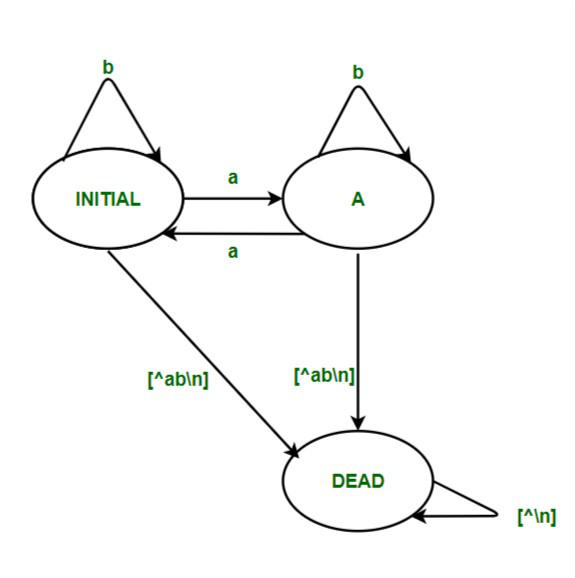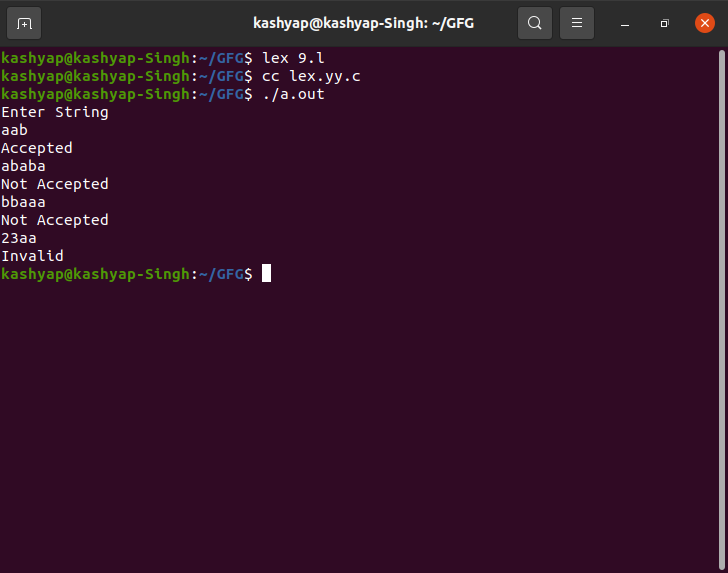# LEX Code that accepts string having even number’s of ‘a’ over input alphabet {a,b}

• Difficulty Level : Hard
• Last Updated : 26 Jul, 2021

Prerequisite :  Designing Finite Automata

Introduction :
In this article, we will discuss the DFA in LEX Code that accepts the string having even number’s of ‘a’ over input alphabet  {a, b}. with the help of example. Let’s discuss it one y one.

Problem Overview :
LEX Code that accepts the string having even number’s of ‘a’ over input alphabet  {a, b}.

Example –

```Input : aba
Output: Accepted

Input : ababba
Output: Not Accepted

Input: 23ab
Output:Invalid

Input:ab345
Output:Invalid

Input:aabababa
Output:Not Accepted```

Approach :
LEX provides us with an INITIAL state by default. So to make a DFA, use this as the initial state of the DFA.
We define two more states – A  and DEAD, where the DEAD state would be used if encountering a wrong or invalid input. When the user inputs an invalid character, move to DEAD state, and then print “Invalid”.
If the input string ends at A then display the message “Not Accepted”. Else if the input string ends at state INITIAL, then displays the message “Accepted”.Note :
To compile the lex program we need to have a Unix system that has flex installed into it. Then we need to save the file with the .l extension.

`Example - filename.l`

Then after saving the program closes the Lex file and then open the terminal and write the following commands as follows.

```lex filename.l
cc lex.yy.c
./a.out```

LEX CODE :

```%{
%}

%%
<INITIAL>a BEGIN A;
<INITIAL>b BEGIN INITIAL;
<INITIAL>\n BEGIN INITIAL; {printf("Accepted\n");}

<A>a BEGIN INITIAL;
<A>b BEGIN A;
<A>\n BEGIN INITIAL; {printf("Not Accepted\n");}

<DEAD>\n BEGIN INITIAL; {printf("Invalid\n");}

%%

int yywrap()
{
return 1;
}
int main()
{
printf("Enter String\n");
yylex();
return 0;
}```

OUTPUT –My Personal Notes arrow_drop_up